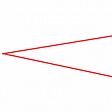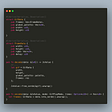# Implementing the Mini-Language Arith in Elm

In my quest to better understand type theory, I’ve started reading Benjamin Pierce’s excellent book, Types and Programing Languages. Early on he introduces a little language — `arith`. While it is an untyped language, it is a superb vehicle for illustrating many fundamental notions and tools, e.g., structural induction, abstract syntax trees, etc. What I would like to describe here is a parser and interpreter for this language written in Elm. Writing code for these is a little exercise that helps to understand better some of the ideas in the book. Pierce gives a link to parser and interpreter code in OCaml. But of course one learns more if one does it oneself. The code for the Elm implementation is on GitHub.

Note (May 6, 2020): I’ve added an article on type-checking `Arith`.

## The Grammar of Arith

The language has a very simple grammar sufficient to express some of the structure of the natural numbers. Simple foundations for the natural numbers were formulated by Peano (1879). A key feature of his formulation was the successor function succ: given a natural number n, the next natural number is succ n.

To describe the grammar of `arith`, we give rules for forming its terms: (1) the symbols `true`, `false`, and 0 are terms; (2) if `t` is a term, then so are `succ t`, `pred t`, and `iszero `t; (3) If `s` `t` and `u` are terms, then so is `if t then s else u`. Here is an example of a “sentence” in `arith`, as a human would read it or write it:

`   S = if iszero succ 0 then succ succ succ 0 else false`

The real structure of the sentence S is given by the tree T pictured in the figure on the left. As we shall see shortly, one can construct a function `parse` that maps sentences `s` to one of two possible values. The first, in the case where the sentence "makes sense,” is`Ok t`, where `t` is a representation of the tree corresponding to `s`. The second value, in the case where `parse` does not understand `s`, is `Err str`, where `str` is a string representing an error message. There is also a function `stringValue`, which maps trees to strings. These two functions are, roughly speaking inverses of each other. Therefore the string and tree representations of a term of `arith` are equivalent. Note, by the way, that there is no need for parentheses in the s-representation. The grammar is sufficient to give it an unambiguous meaning.

The tree created by `parse` is called an abstract syntax tree, or AST, for short. Jumping ahead a bit, let’s test the functions `parse` and `stringValue` using the Elm repl. We start with a string `input`, apply `parse` to it to produce an AST, then apply `stringValue` to the AST to recover the string `input` :

`> input = "if iszero succ 0 then succ succ succ 0 else false"> parse input  Ok (IfExpr (IsZero (Succ Zero)) (Succ (Succ (Succ Zero))) F)  : Result (List Parser.DeadEnd) Term> ast = IfExpr (IsZero (Succ Zero)) (Succ (Succ (Succ Zero))) F> stringValue ast"if iszero succ 0 then succ succ succ 0 else False" : String`

If the input is nonsense, then the parser returns an error value:

`> parse "if 0 then pred else succ"  Err [...]  : Result (List Parser.DeadEnd) Term`

## The AST

We said that `ast` is the abstract syntax tree corresponding to the input sentence. We picture a representation of this tree on the left; it is a two-dimensional structure. But what we see in the terminal, is a “linear,” one-dimensional structure, with one word after another, albeit including parentheses. Both are representations of an Elm value. Every Elm value has a type, so we must formulate a definition of the type of AST’s, or what is the same thing, of terms. This is what we do next.

## The Type of a Term

Define a custom type (aka algebraic data type):

`type Term    = T    | F    | Zero    | Succ Term    | Pred Term    | IsZero Term    | IfExpr Term Term Term`

Notice that definition of `Term` is recursive, since it refers to itself. Note also the very close, essentially one-to-one correspondence between the definition of `Term` and the rules of the grammar.

## A Parser

Our next task is to construct a parser—a function `parse`that will, if successful, map strings to values of type `Term`:

`parse : String -> Result (List Parser.DeadEnd) Termparse str = Parser.run term str`

Note the type for the function value, `Result error value`. It enables us to write functions which may succeed or fail, which in the case at hand means parse successfully or fail to parse. The function `Parser.run` (from the elm/parser library) “runs” a `Parser` on its input. Thus the real task is to understand the parser `term`, defined below. (We will explain things here modulo more detail to be found in th elm/parser library and in the code on GitHub).

`term : Parser Termterm =    succeed identity        |. spaces        |= oneOf            [ true            , false            , zero            , succ            , pred            , iszero            , lazy (\_ -> ifExpr)            ]`

The parser `term` first “eats” any spaces it encounters, then uses the `oneOf` parser to try a list of parsers, each one in turn. If it succeeds with a component parser, it stops and returns the value parsed. If it succeeds with no component parser it returns an error value. Let us examine the component parsers. Those like the `true` parser are easy to understand:

`true : Parser Termtrue =    succeed T        |. symbol "true"`

If the string “true” can be eaten by the parser, the value `T : Term` is returned. Parsers like `succ` are slightly more complicated:

`succ : Parser Termsucc =    symbol "succ"        |> andThen (\_ -> term)        |> map (\t -> Succ t)`

The idea is that this parser first tries to eat the string “succ”. If successful, the parser then runs the parser`term` to recognize the term`t` in `suc t`. If that operation is successful, the term recognized is wrapped with the `Term` `Succ`by applying the function `\t -> Succ t`. To really understand what is going on here, one needs to work out all of the types, making sure that they fit together. For example, consulting the elm/parser library, we find that

`andThen : (a -> Parser b) -> Parser a -> Parser b`

Thus `andThen` gives (roughly speaking), a way to follow one parser with another, hence a way to sequence parsers where the second parser can receive information from the first. In more detail, using `andThen`, one can combine a `Parser a` with a function `a -> Parser b` to produce value of type `Parser b`. In the case above, the first parser (`Parser a`), is `symbol "succ"` and the second is `term` . We use the function `\t -> term` because no argument to `term` is needed.

Finally, there is the parser for inputs like `if true then false else succ 0`. It uses a parser pipeline which operates as follows: (a) recognize the symbol “if”, then a term; (b) eat spaces, then the symbol “then”, then a term; c) eat spaces, the symbol “else”, then a term. The effect of the parser `(|.)` is to run the parser on its right, but to ignore the value parsed. The effect of the parser `(|=)` is similar, except that the value parsed is kept. In the end, all the “kept” values are fed as arguments to the function `IfExpr` to construct a value of type `Term`.

`ifExpr : Parser TermifExpr =    succeed IfExpr        |. symbol "if"        |= term        |. spaces        |. symbol "then"        |= term        |. spaces        |. symbol "else"        |= term`

The use of `lazy` as in . `lazy (_ -> ifExpr)` is explained by the following remark (cf Czapliki):

In Elm, you can only define a value in terms of itself it is behind a function call. So `lazy` helps us define these self-referential parsers. (`andThen` can be used for this as well!)

## An Evaluator

In this section, we discuss two functions, `eval : Term -> Value` and `evalString : String -> Value`. The second, which is the interpreter for `arith` is (essentially) the composition `eval o parse`, so the real work is in defining `eval`.

To define `eval`, we must first decide what is the type of the values it computes:

`type Value    = Numeric Int    | Boolean Bool    | Error String`

The definition accounts for the fact that there could be either numeric values, like `succ succ 0`, otherwise known as `2`, or the Boolean values `True` or `False`. It also accounts for the fact that evaluation may fail. For example, the string

`input = "if succ 0 then 0 else succ 0"`

can be parsed into a valid AST, namely

`ast = IfExpr (Succ Zero) Zero (Succ Zero)`

However, evaluation fails, because the first argument of an if-expression must be a Boolean:

`> eval astError ("If-then-else: expecting boolean value") : Value`

Below is the definition of `eval`. It depends on (and expresses) some reasonable (but here unwritten) semantics for the language `arith`. The function `eval`is a big `case` statement, with one branch for each part of the definition of `Term`.

`eval : Term -> Valueeval t =    case t of        Zero ->            Numeric 0        Succ a ->            case eval a of                Numeric b ->                    Numeric (b + 1)                _ ->                    Error "succ expects numeric value"        Pred a ->            case eval a of                Numeric b ->                    if b == 0 then                       Numeric 0                     else                       Numeric (b - 1)                _ ->                    Error "pred expects numeric value"        F ->            Boolean False        T ->            Boolean True        IsZero a ->            case eval a of                Numeric b ->                    Boolean (b == 0)                _ ->                    Error "iszero expects a numeric value"       IfExpr a b c ->            case eval a of                Boolean v ->                    case v of                        True ->                            eval b                        False ->                            eval c                _ ->                     Error "If-then-else: expecting boolean value"`

1. The reason that the `eval` function can produce values of type `Error String` is that the language `arith` is not typed. Thus sentences such as “if succ iszero succ 0 then 0 else succ 0” can be compiled into a valid AST but cannot be evaluated without error.
2. Julian Antonielli has some very nice slides describing a type checker for `arith`. His implementation is in Haskell. I plan to give it a try in Elm. Among the references at the end of Julian’s slides is a link to a YouTube video on “typechecking from scratch.”
3. A good exercise is to rig up a function to compute the depth of the AST produced by `parse`. Another good exercise is to work out a definition of `stringValue`. You will find code for both of these in the GitHub repo for the Elm implementation of `arith`.

## Definition of depth

1. The depth of a term like `true`, `false`, `0` is 1.
2. The depth of the term `succ t` is 1 + depth `t`, and similarly for `pred` and `iszero`.
3. The depth of `if a then b else c` is 1 + max(depth a, depth b, depth c).

## More from James Carlson

jxxcarlson on elm slack, http://jxxcarlson.github.io

Love podcasts or audiobooks? Learn on the go with our new app.

## The most useful shortcuts I’ve ever used in Office## How to Scan whole country IP Addresses in a while## Using Moq for Unit Testing with Prism EventAggregator## Describing a project on EasyEDA## James Carlson

jxxcarlson on elm slack, http://jxxcarlson.github.io

## Avoiding using Serde in Rust WebAssembly When Performance Matters